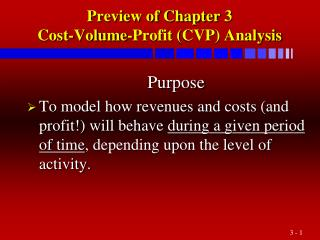# Preview of Chapter 3 Cost-Volume-Profit ( CVP ) Analysis - PowerPoint PPT PresentationDownload PresentationPreview of Chapter 3 Cost-Volume-Profit ( CVP ) Analysis

Preview of Chapter 3 Cost-Volume-Profit ( CVP ) AnalysisDownload Presentation## Preview of Chapter 3 Cost-Volume-Profit ( CVP ) Analysis

- - - - - - - - - - - - - - - - - - - - - - - - - - - E N D - - - - - - - - - - - - - - - - - - - - - - - - - - -
##### Presentation Transcript

1. Preview of Chapter 3Cost-Volume-Profit (CVP) Analysis Purpose • To model how revenues and costs (and profit!) will behave during a given period of time, depending upon the level of activity.

2. CVP Model • Assumes a contribution margin income statement: Contribution Mgn ApproachvsAbsorption Costing Sales 100% Sales - Variable Costs: - VC% - CGS (Var. CGS, Selling, Admin.) = Gross Margin = Cont. Margin = CM% - Period Costs - Fixed Costs: (Selling, Admin.) (Mfg., Selling, Admin.) Operating Income Operating Income • Same only if inventories are constant (production = sales).

3. Questions Besides “Where’s the breakeven point,” other questions are of more interest: • How will the BEP increase if FC or VC increases? • How much higher could VC/unit rise before we’d have a loss for the period? • How far could sales drop below the forecast before Operating Income would fall below last year’s?

4. Equations For CVP Analysis • Graphs are for exposition only. We must solve using equations. • A “definitional” equation, defining income: Sales – Var Cost – Fixed Cost = Operating Income • Good starting point to attack unusual CVP questions

5. Example of Relationships For a particular item, Unit Price \$2.50 100% Unit VC 1.7570% (VC%) Unit CM \$ .75 30% (CM%)

6. Equations For CVP Analysis • Recognizing that VC and CM are % of sales: Sales – (VariableCost%)Sales – FixedCost= Operating Income Contribution Margin {OR} Sales x (CM%) – FC = Operating Income CM If FC = \$10,000, how many must we sell to BE? S - .7S – 10,000 = 0 .3S = 10,000 S = \$33,333 [÷ \$2.50 = 13,333 units]

7. Other Handy Equation Forms • Sales Dollars = (FC + Oper. Inc.) / CM% • Units Sold = (FC + Oper. Inc.) / (CM per unit)

8. Wide Applicability of CVP • CVP applies to any question about proposed changes in cost structures and related volume effects. • Widely applicable. • Assigned problems are representative.

9. Product-Mix Problem PRODUCT A B C Price \$10 \$15 \$25 The Weighted VC 8 7 10 Average CM% CM \$ 2 \$ 8 \$15 will depend on actual mix sold CM% 20% 53.33% 60%

10. Product-Mix Problem Weighted Average based on previous year’s results (assumed numbers): A B C Tot.WtdAvg Units Sold 5000100001500030000 Sales \$50,000 150,000 375,000 575,000 1.000 VC 40,00070,000150,000260,000 .452 CM 10,000 80,000 225,000 315,000 .548

11. Product-Mix Problem • What’s wrong with the following approach? Product mix is 5/30 “A”, 10/30 “B” and 15/30 “C” So 5/30 x .20 + 10/30 x .533 + 15/30 x .60 = .511 ≠ .548 • Error: Done in terms of units, but the CM% is contribution/\$, not contribution/unit! Correct: (50/575)(.20) + (150/575)(.533) + (375/575)(.60) = .548

12. Considering Income Tax • Recall the “definitional” equation, defining income: Sales – Var Cost – Fixed Cost = Operating Income (1-r)*(Sales – Var Cost – Fixed Cost) = Income after tax Sales – Var Cost – Fixed Cost = (Income after tax)/ (1-r) So, divide desired after-tax income by (1-r) to get the desired before-tax income and use the formulas as usual.

13. Effect of Income Taxes • Any amount “after tax” or net of taxes = (1-r) (The amount before taxes) [Applies to an expense, revenue, or Operating Income] • \$1,000 expense is tax deductible So at 40% rate: (1-.4) 1,000 = \$600 net expense • \$1,000 revenue is taxable So at 40% rate: (1-.4) 1,000 = \$600 net

14. Effect of Income Taxes • All numbers in the CVP equations are before tax. Therefore questions involving “after tax” effects require you to convert to “before tax” before using the equation. • Ex. How many units sold to earn \$900,000 after tax at a 45% tax rate? AT amt. = (1-r) BT amt. 900,000 = (1-.45) BT amt. BT amt. = 900,000/.55 = \$1,636,364 • Thus, using my Eq. 4: Units = FC + 1,636,364 CM/unit